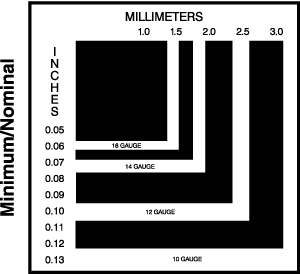# Metric Conversion Information

#### Length

 1 mm = 0.039 in. 8 mm = 0.315 in. 60 mm = 2.362 in. 400 mm = 15.748 in. 2 mm = 0.079 in. 9 mm = 0.354 in. 70 mm = 2.756 in. 500 mm = 19.685 in. 3 mm = 0.118 in. 10 mm = 0.394 in. 80 mm = 3.150 in. 600 mm = 23.622 in. 4 mm = 0.157 in. 20 mm = 0.787 in. 90 mm = 3.543 in. 700 mm = 27.559 in. 5 mm = 0.197 in. 30 mm = 1.181 in. 100 mm = 3.937 in. 800 mm = 31.496 in. 6 mm = 0.236 in. 40 mm = 1.575 in. 200 mm = 7.874 in 900 mm = 35.433 in. 7 mm = 0.276 in. 50 mm = 1.969 in. 300 mm = 11.811 in. 1000 mm = 39.370 in. Imperial to Metric Metic to Imperial 1 inch = 25.40 mm 1 foot = 0.3048 m 1 mm = 0.03937 in. 1 m = 3.281 feet 1 inch = 2.54 cm 1 yard = 91.44 cm 1 cm = 0.3937 in. 1 m = 1.0936 yards 1 foot = 30.48 cm 1 yard = 0.9144 m 1 m = 39.37 in.

#### Area

 1 sq. inch = 645.16 sq. millimeters 1 sq. millimeter (mm2) = 0.00155 sq. inch 1 sq. inch = 6.4516 sq. centimeters 1 sq. centimeter (cm2) = 0.1550 sq. inch 1 sq. foot = 929.03 sq. centimeters 1 sq. meter (m2) = 10.7640 sq. feet 1 sq. foot = 0.0929 sq. meter 1 sq. meter (m2) = 1.196 sq. yards 1 sq. yard = 0.836 sq. meter

#### Temperature

Imperial to Metric
Absolute Units
1° Fahrenheit = 0.555° Celsius
To convert a °F temperature reading, use the following equation:
(°F - 32) x 0.555 = °C

Metric to Imperial
Absolute Units
1° Celsius = 1.8° Fahrenheit